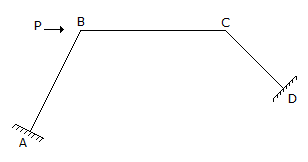# Civil Engineering - GATE Exam Questions

### Exercise :: GATE Exam Questions - Section 4

31.

For the determination of earth pressure, Coulomb's wedge theory assumes that:

 A. the back of wall is smooth and vertical B. the soil is non-homogeneous and anisotropic C. the slip surface is circular D. the wall surface is rough

Explanation:

No answer description available for this question. Let us discuss.

32.

The degree of kinematic indeterminacy of the rigid frame with clamped ends at A and D shown in the below figure is,A. 4 B. 3 C. 2 D. zero

Explanation:

No answer description available for this question. Let us discuss.

33.

The dimensions for the flexural rigidity of a beam element in mass (M), length (L) and time (T) is given by

 A. MT-2 B. ML3T-2 C. ML-1T-2 D. M-1T2

Explanation:

No answer description available for this question. Let us discuss.

34.

The centre of pressure of a liquid on a plane surface immersed vertically in a static body of liquid, always lies below the centroid of the surface area, because

 A. in liquids the pressure acting is same in-all directions B. there is no shear stress in liquids at rest C. the liquid pressure is constant over depth D. the liquid pressure increases linearly with depth

Explanation:

No answer description available for this question. Let us discuss.

35.

The most commonly used sampler for obtaining a disturbed sample of the soil is

 A. split spoon sampler B. open drive sampler C. piston sampler D. thin wall shelby tube sampler